# Dirichlet's Unit Theorem

In this chapter we will prove the main structure theorem for the group of units of the ring of integers of a number field. The answer is remarkably simple: if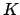has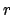real andcomplex embeddings, then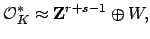where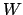is the finite cyclic group of roots of unity in. Examples will follow on Thursday (application: the solutions to Pell's equation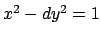, for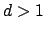squarefree, form a free abelian group of rank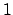).

Subsections

William Stein 2004-05-06